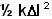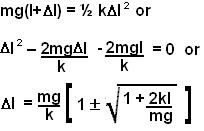## Monday, May 5, 2008

### Irodov Problem 1.139The figure depicts what happens in the problem. The mass m starts its journey from the top and slides downwards until it reaches the end of the rubber cord. At this point the mass has gained a lot of momentum and so when it hits the end it elongates the rubber cord with its impact and weight by a lengthmaking the total length. The question asks the value of.

The problem can be easily solved by using conservation of energy. The rubber cord exerts a restoring force ofwhen the rubber band is elongated by(similar to a spring) and the energy stored in the rubber cord will be. At this point the mass has fallen a height ofand thus lost a potential energy of. Also the mass would have stopped moving at this point so it has no kinetic energy. Since energy is always conserved the potential energy lost by the mass must be now stored in the elongated rubber cord. In other words,If the mass m were not dropped and simply placed at the bottom of the rubber cord, it would elongate the rubber cord by a length of mg/k - this would be the equilibrium point of the rubber cord with mass. Since it was dropped from a height, the mass will exhibit simple harmonic motion about the equilibrium point. The + and - signs in the solution represent the maximum and minimum elongations experienced by the rubber cord during this simple harmonic motion. The maximum elongation is thus simply the solution with the + sign.